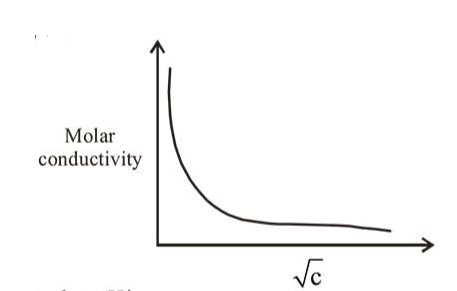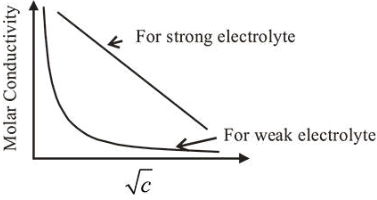# The variation of molar conductivity with concentration of an electrolyteQuestion:

The variation of molar conductivity with concentration of an electrolyte $(X)$ in aqueous solution is shown in the given figure.The electrolyte $X$ is :

1. HCL

2. NaCL

3. $\mathrm{KNO}_{3}$

4. $\mathrm{CH}_{3} \mathrm{COOH}$

Correct Option: , 4

Solution:Among given electrolytes, $\mathrm{CH}_{3} \mathrm{COOH}$ is weak electrolyte .